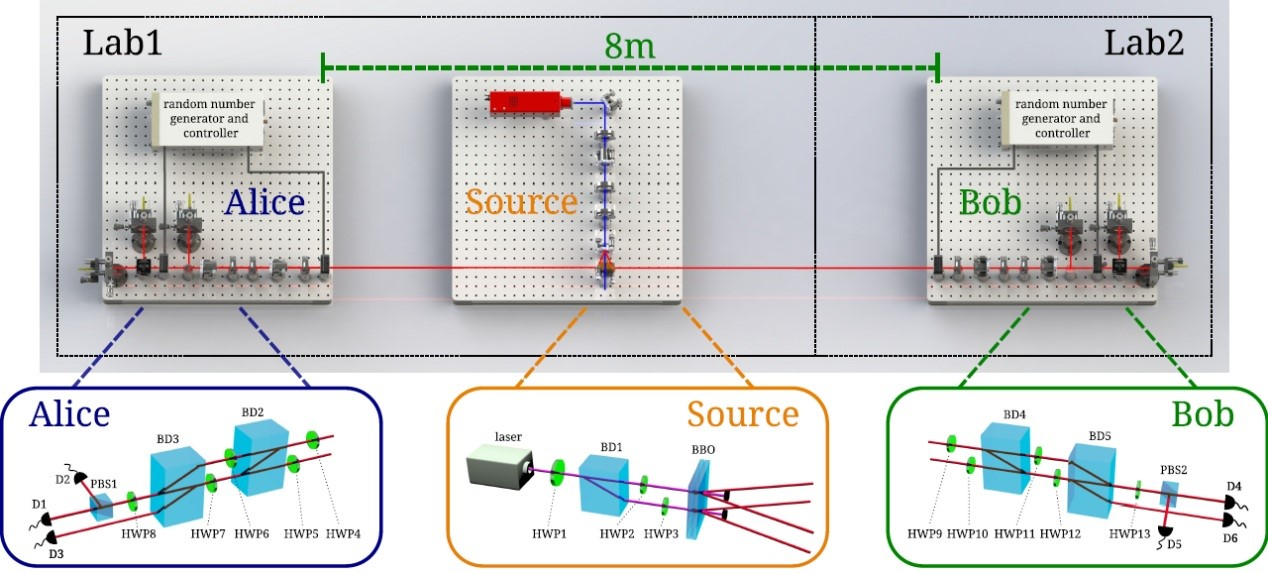• more
• #### Scientists Observe Stronger-than-Binary Correlations with Entangled Photonic Qutrits

•[2018-05-07]
• Prof. Chuan-Feng Li’s Group of CAS Key Laboratory of Quantum Information has made new progress in the study of fundamental problems in quantum mechanics. Chuan-Feng Li, Bi-Heng Liu, and others collaborated with theoretical physicists from Spain, Hungary, and Germany to demonstrate for the first time the existence of stronger-than-binary correlations in quantum mechanics. The results were published in Physical Review Letters on May 4th and were incorporated into the Editor's Suggestion.

Let's look at the measurement problem. Quantum measurement is one of the basic problems in quantum mechanics. In classical theory, complex multivalued problem measurements can be disassembled into binary measurements. For example, for a three-outcome problem (set the output results to 1, 2, and 3, respectively), two-step two-outcome measurement can be used to complete: The first step is to determine whether the measurement result is 1, if not, then proceed to the next two-outcome (2 3) Measurement to determine if the measurement is 2 or 3. However, for quantum system measurements, things are not so simple. The theoretical collaborators of the research group used the three-dimensional quantum entanglement to construct a special inequality based on the binary theory. The violation of the inequality means the existence of stronger-than-binary correlations in Quantum Mechanics. However, it is very difficult to test this inequality experimentally because of the need for extremely high-quality sources of high-dimensional entanglement.

As early as 2016, Li’s group developed high-quality three-dimensional entangled photon sources [PRL 117, 170403 (2016)]. To test this inequality based on binary theory, they simplified the design of the entangled photon source and further improved the performance of the entangled photon source. In Experiment, they distributed the two photons entangled in three dimensions to two laboratories 8 meters apart and measured them with randomly chosen measurement bases. The experimental observation violates the inequality with more than 9 standard deviations and demonstrates for the first time the existence of stronger-than-binary correlations in quantum mechanics.

They have published the complete original data of this experiment online for international peers to conduct more in-depth data analysis. This experiment will promote further research on the fundamental problem of quantum theory.

https://journals.aps.org/prl/abstract/10.1103/PhysRevLett.120.180402Setup of the experiment